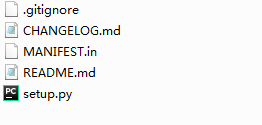# 从文件中读取数据

``f.read(size)``

``f.readline()``

``f.readlines()``

mode 解释
r 以只读方式打开
w 以写方式打开一个文件，当这个文件存在时，覆盖原来的内容。当这个文件不存在时，创建这个文件
x 创建一个新文件，以写方式打开，当文件已存在， 报错FileExistsError
a 以写方式打开，写人内容追加在文件的末尾
b 表示二进制文件，添加在其他控制字符后
t 表示文本文件，默认值
+ 以修改方式打开，支持读写

# 设置工作目录

• 获取当前工作目录：
``````import os
os.getcwd(' ')    ``````
• 设置工作目录
``os.chdir(' ') ``

# 设置`Jupyter Notebook`图片输出格式

• SVG格式

• Jupyter Notebook魔术命令

``````%matplotlib inline
%config InlineBackend.figure_format = 'svg'``````

# 让图片说中文

``````import matplotlib.pyplot as plt
plt.rcParams['font.sans-serif']=['SimHei']
plt.rcParams['axes.unicode_minus']=False``````

# Jupyter Notebook 更换主题背景

``jt -t monokai -f roboto -nf robotosans -tf robotosans -N -T -cellw 70% -dfs 10 -ofs 10``

``jt -t grade3``

# 异常处理

``````a=3
b=2
for i in range(5):
try:
a = a - 1
c=b/a
print(c)

except Exception as e:
print(e)#输出异常行为名称``````
``````1.0
2.0
division by zero
-2.0
-1.0``````

# Anaconda 或Python 从本地离线安装第三方包

1. 首先从github上clone或者直接下载zip文件并解压到目录A。

2. 目录A下有setup等文件：1. 如果是windows系统，在目录A下，在未选择任何文件的情况下(可以先左键单击空白处)按住Shift的同时在空白处单击右键，调出菜单。此时点击【在此处打开命令窗口】的选项。

2. 在弹出的命令窗口中输入：

``python setup.py install``
1. 安装完成!

# 在R Studio中运行Python

Python和R都是数据科学的必备工具。熟悉R的人都知道，R Studio是一款非常出色的R语言IDE。但一直以来，Python和R都是割裂的。`reticulate`包能够为我们提供一些Python的调用功能。包括两个语言中对象的相互转化。

``````nb_rmd = rmarkdown:::convert_ipynb(nb_file)
xfun::file_string(nb_rmd)
rmarkdown:::convert_ipynb("XXX.ipynb",
output = "YYY.Rmd")``````